# Examination Management System in C

• Last Updated : 28 Feb, 2022

Problem Statement: Write C program to build a Software for Examination Management System that can perform the following operations:

• Add/Delete the Details of the Students
• Attendance Monitoring of the students
• Set/Edit Eligibility criteria for exams
• Check Eligible Students for Exams
• Print all the records of the students

Approach: The idea is to form an individual function for each operation. All the functions are unified together with switch cases to form software. Below is the illustration of the functions:

1. execute(): This function will shows the available choices for the software and will perform the below functionality using Switch Statements
Functionality:
• Show Eligible Students
• Delete Student Record
• Update Eligibility Criteria
• Print Details of Students
2. add(): This function that get the data from the user and update the list of the students. While adding the student into the list, check for the uniqueness of the Roll Number of the student. Details of the students to be added are:
• Name of Student
• Roll Number
• Fees Status
• Attendance Record of student
3. eligibleStudents(): This function shows the previous attendance percentage required for exams and get the data from the user and update the eligibility for the exams. It also updates the fee status required for the eligibility of exams by iterating over the List of the student records and for every student check the attendance percentage is above the percentage required and fee status of the student.

4. print_student(): This function iterate over the list of students and print the details of the student.

5. delete(): This function get the student roll number to delete the student record and update the student’s list.

Below is the program to illustrate the above functionalities:

``````
// Function that adds the student's
// record in Examination Management
// Project
{
printf("Enter the total ");

printf("number of working days \n");
scanf("%f", &tdays);

printf("Enter the number"
"of students \n");
scanf("%d", &n);

for (i = 0; i < n; i++) {

printf("Student number %d \n",
(i + 1));

printf("Enter the name of"
" the student \n");
scanf("%s", s[i].name);

printf("Enter the roll number \n");
scanf(" %d", &s[i].rno);

printf("Enter the fees of the"
"student 'P' for paid "
", 'N' for not paid \n");
scanf(" %c", &s[i].fees);

printf("Enter the number of"
"days the student was "
"present \n");
scanf("%f", &s[i].days);

s[i].attend = (s[i].days
/ tdays)
* 100;

printf("student attendance"
" = %f \n",
s[i].attend);
}
execute();
}
``````

execute()

``````
// Function to execute the Examination
// Management System
void execute()
{
printf("Enter the serial number "
"to select the option \n");
printf(" 1. To show Eligible"
"candidates \n");
printf(" 2. To delete the "
"student record \n");
printf(" 3. To change the "
"eligibility criteria \n");
printf(" 4. Reset the "
"eligibility criteria \n");
printf(" 5. Print the list "
"of all the student \n");
printf(" Enter 0 to exit \n");
scanf("%d", &option);

// Switch Statement for choosing
// the desired option for user
switch (option) {
case 1:
eligibleStudents(s);
execute();
break;

case 2:
deleteRecord(s);
execute();
break;

case 3:
printf("Old Attendance "
"required = %f",
present);
printf(
"\n Enter the updated "
"attendence required \n");
scanf("%f", &present);
printf("fees status required"
" was %c \n",
money);
printf("Enter the new fees "
"status 'P' for paid 'N' "
"for not paid and "
"'B' for both \n");
scanf("%c", &money);

printf("Eligibility Criteria"
" updated \n");
execute();
break;

case 4:
present = 75.00;
money = 'P';
printf("Eligibility Criteria"
" reset \n");
execute();
break;

case 5:
printStudents(s);
execute();
break;

case 6:
execute();
break;

case 0:
exit(0);

default:
printf("Enter number only"
" from 0 - 4 \n");
execute();
}
}
``````

eligibleStudents()

``````
// Function to print the student
// details of the eligible students
void eligibleStudents(struct student s[])
{
printf("________________"
"________________"
"_______________"
"_____________ \n");
printf("Qualified student are = \n");

// Iterate over the list of the
// students records
for (i = 0; i < n; i++) {
// Condition to check the
// eligibility of the student
if (s[i].fees == money || 'B' == money) {
if (s[i].attend >= present) {
printf("Student name = %s \n",
s[i].name);

printf("Student roll no. = %d \n",
s[i].rno);

printf(" Student fees = %c \n",
s[i].fees);

printf(" Student attendence = %f \n",
s[i].attend);
}
}
}
}
``````

delete()

``````
// Function to delete any Student Record
void delete (struct student s[])
{
int a = 0;
printf("Enter the roll number of"
"the student to delete it ");
scanf("%d", &a);

// Iterate over the student's
// records to delete the Data
for (i = 0; i <= n; i++) {
// Check the current student
// roll number is same as the
// user input roll number
if (s[i].rno == (a)) {

// Update record at ith index
// with (i + 1)th index
for (j = i; j < n; j++) {
strcpy(s[j].name,
s[j + 1].name);

s[j].rno = s[j + 1].rno;
s[j].fees = s[j + 1].fees;
s[j].days = s[j + 1].days;
s[j].attend = s[j + 1].attend;
}
printf("Student Record deleted");
}
}
}
``````

print_student()

``````
// Function to print the students record
void print_student(struct student s[])
{
// Iterate over the students
// students records
for (i = 0; i < n; i++) {
printf("Name of student %s \n",
s[i].name);
printf("Student roll number = %d \n",
s[i].rno);
printf("Student fees status = %c \n",
s[i].fees);
printf("student number of days "
"present = %d \n",
s[i].days);
printf("Student attendence = %f \n",
s[i].attend);
}
}
``````

## Complete Code

 `// C program for the Examination``// Management System``#include ``#include ``#include ` `int` `option = 0;``int` `i = 0;``int` `n = 0;``int` `j = 0;``float` `present = 75.00;``char` `money = ``'P'``;``float` `tdays = 1;` `// Structure of Student``struct` `student {``    ``char` `name;``    ``int` `rno;``    ``char` `fees;``    ``float` `days;``    ``float` `attend;``} s;` `// Functions``void` `add(``struct` `student s[]);``void` `eligibleStudents(``struct` `student s[]);``void` `execute();``void` `printStudents(``struct` `student s[]);``void` `deleteRecord(``struct` `student s[]);` `// Function to execute the software``// for the student examination``// registration system``void` `execute()``{``    ``printf``(``        ``" Enter the serial number"``        ``"to select the option \n"``);``    ``printf``(``" 1. To show Eligible"``           ``"candidates \n"``);``    ``printf``(``" 2. To delete the "``           ``"student record \n"``);``    ``printf``(``" 3. To change the "``           ``"eligibility criteria \n"``);``    ``printf``(``" 4. Reset the "``           ``"eligibility criteria \n"``);``    ``printf``(``" 5. Print the list "``           ``"of all the student \n"``);``    ``printf``(``" Enter 0 to exit \n"``);` `    ``scanf``(``"%d"``, &option);` `    ``// Switch Statement for choosing``    ``// the desired option for the user``    ``switch` `(option) {``    ``case` `1:``        ``eligibleStudents(s);``        ``execute();``        ``break``;` `    ``case` `2:``        ``deleteRecord(s);``        ``execute();``        ``break``;` `    ``case` `3:``        ``printf``(``"Old Attendance "``               ``"required = %f"``,``               ``present);``        ``printf``(``            ``"\n Enter the updated "``            ``"attendence required \n"``);``        ``scanf``(``"%f"``, &present);``        ``printf``(``"fees status required"``               ``" was %c \n"``,``               ``money);``        ``printf``(``"Enter the new fees "``               ``"status 'P' for paid 'N' "``               ``"for not paid and "``               ``"'B' for both \n"``);``        ``scanf``(``"%c"``, &money);``        ``printf``(``"Eligibility Criteria updated \n"``);``        ``execute();``        ``break``;` `    ``case` `4:``        ``present = 75.00;``        ``money = ``'P'``;``        ``printf``(``"Eligibility creitria reset \n"``);``        ``execute();``        ``break``;` `    ``case` `5:``        ``printStudents(s);``        ``execute();``        ``break``;` `    ``case` `6:``        ``execute();``        ``break``;` `    ``case` `0:``        ``exit``(0);` `    ``default``:``        ``printf``(``"Enter number only from 0-4 \n"``);``        ``execute();``    ``}``}` `// Function to print the students record``void` `printStudents(``struct` `student s[])``{``    ``// Loop to iterate over the students``    ``// students records``    ``for` `(i = 0; i < n; i++) {` `        ``printf``(``"Name of student %s \n"``,``               ``s[i].name);``        ``printf``(``"Student roll number = %d \n"``,``               ``s[i].rno);``        ``printf``(``"Student fees status = %c \n"``,``               ``s[i].fees);``        ``printf``(``"Student number of days "``               ``"present = %f \n"``,``               ``s[i].days);``        ``printf``(``"Student attendence = %f \n"``,``               ``s[i].attend);``    ``}``}` `// Function to Student Record``void` `deleteRecord(``struct` `student s[])``{``    ``int` `a = 0;``    ``printf``(``"Enter the roll number of "``           ``"the student to delete it "``);``    ``scanf``(``"%d"``, &a);` `    ``// Loop to iterate over the students``    ``// records to delete the Data``    ``for` `(i = 0; i <= n; i++) {``        ``// Condition to check the current``        ``// student roll number is same as``        ``// the user input roll number``        ``if` `(s[i].rno == (a)) {` `            ``// Update record at ith index``            ``// with (i + 1)th index``            ``for` `(j = i; j < n; j++) {``                ``strcpy``(s[j].name, s[j + 1].name);``                ``s[j].rno = s[j + 1].rno;``                ``s[j].fees = s[j + 1].fees;``                ``s[j].days = s[j + 1].days;``                ``s[j].attend = s[j + 1].attend;``            ``}``            ``printf``(``"Student Record deleted"``);``        ``}``    ``}``}` `// Function to print the student``// details of the eligible students``void` `eligibleStudents(``struct` `student s[])``{``    ``printf``(``"________________"``           ``"________________"``           ``"_______________"``           ``"_____________ \n"``);``    ``printf``(``"Qualified student are = \n"``);` `    ``// Iterate over the list``    ``// of the students records``    ``for` `(i = 0; i < n; i++) {``        ``// Check for the eligibility``        ``// of the student``        ``if` `(s[i].fees == money || ``'B'` `== money) {``            ``if` `(s[i].attend >= present) {``                ``printf``(``"Student name = %s \n"``,``                       ``s[i].name);``                ``printf``(``"Student roll no. = %d \n"``,``                       ``s[i].rno);``                ``printf``(``" Student fees = %c \n"``,``                       ``s[i].fees);``                ``printf``(``" Student attendence = %f \n"``,``                       ``s[i].attend);``            ``}``        ``}``    ``}``}` `// Function to add the students record``void` `add(``struct` `student s)``{``    ``printf``(``"Enter the total "``);``    ``printf``(``"number of working days \n"``);``    ``scanf``(``"%f"``, &tdays);` `    ``printf``(``"Enter the number"``);``    ``printf``(``"of students \n"``);``    ``scanf``(``"%d"``, &n);` `    ``for` `(i = 0; i < n; i++) {` `        ``printf``(``"Student number %d \n"``,``               ``(i + 1));` `        ``printf``(``"Enter the name of"``               ``" the student \n"``);``        ``scanf``(``"%s"``, s[i].name);` `        ``printf``(``"Enter the roll number \n"``);``        ``scanf``(``" %d"``, &s[i].rno);` `        ``printf``(``"Enter the fees of the"``               ``"student 'P' for paid "``               ``", 'N' for not paid \n"``);``        ``scanf``(``" %c"``, &s[i].fees);` `        ``printf``(``"Enter the number of"``               ``"days the student was "``               ``"present \n"``);``        ``scanf``(``"%f"``, &s[i].days);` `        ``s[i].attend = (s[i].days``                       ``/ tdays)``                      ``* 100;``        ``printf``(``"student attendence = %f \n"``,``               ``s[i].attend);``    ``}``    ``execute();``}` `// Driver Code``int` `main()``{``    ``printf``(``"Welcome to Student "``           ``"database registration \n"``);` `    ``printf``(``"Enter 0 to exit \n"``);``    ``printf``(``"Enter 1 to add student"``           ``" record \n"``);` `    ``scanf``(``"%d"``, &option);` `    ``// Switch Statements``    ``switch` `(option) {``    ``case` `0:``        ``exit``(0);` `    ``case` `1:``        ``add(s);``        ``break``;` `    ``default``:``        ``printf``(``"Only enter 0 or 1"``);``        ``execute();``    ``}``    ``return` `0;``}`

Output:
Below is the output of the above functionalities: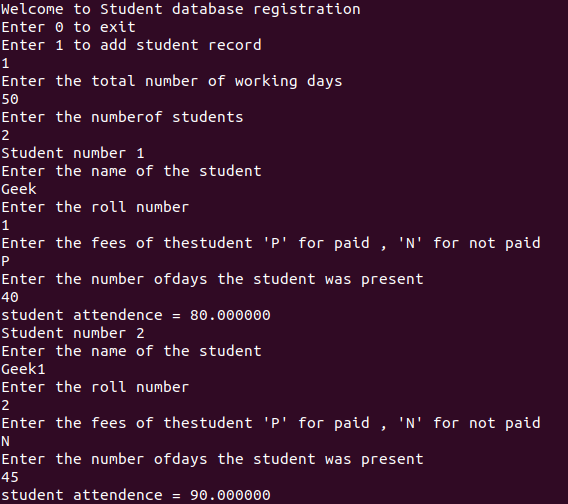• For Eligible Students: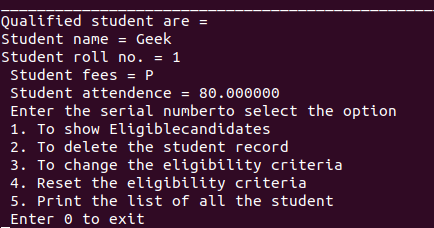• For Eligibility Criteria: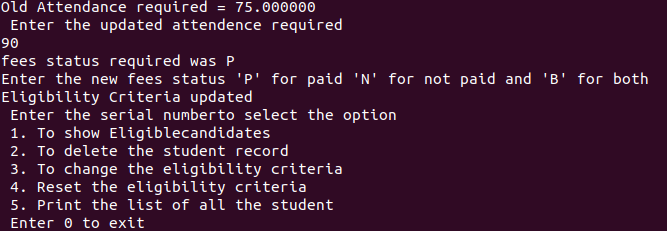• For Printing Student’s Details: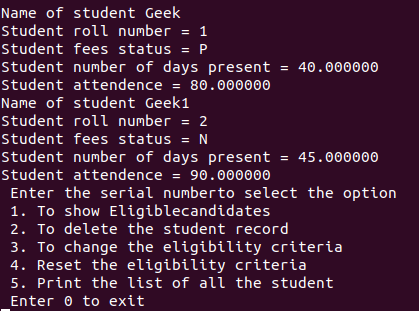• For Deleting Student Record: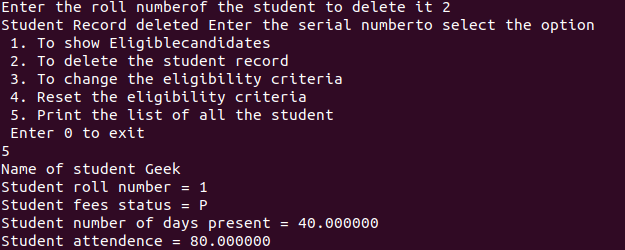My Personal Notes arrow_drop_up